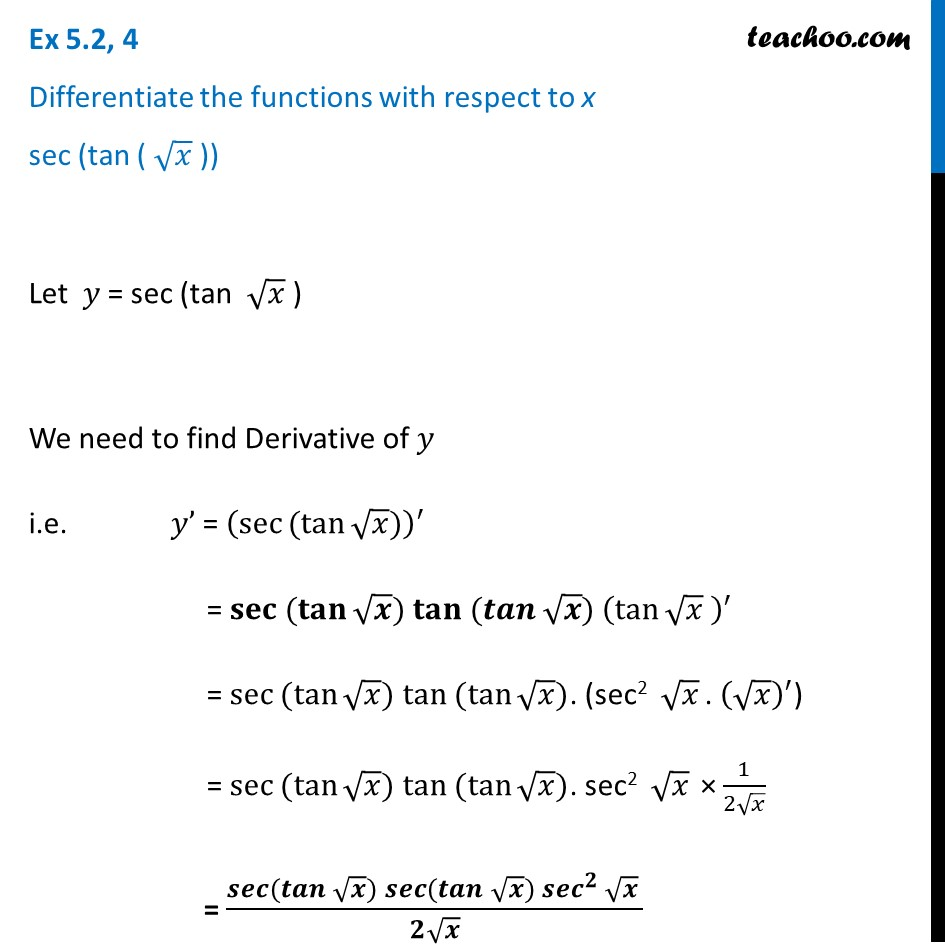Finding derivative of a function by chain rule

Chapter 5 Class 12 Continuity and Differentiability (Term 1)
Concept wise### Transcript

Ex 5.2, 4 Differentiate the functions with respect to x sec (tan ( √𝑥 )) Let 𝑦 = sec (tan √𝑥 ) We need to find Derivative of 𝑦 i.e. 𝑦’ = (sec⁡〖〖(tan〗⁡√𝑥)〗 )^′ = 〖𝐬𝐞𝐜 〗⁡〖(𝐭𝐚𝐧⁡√𝒙)〗 〖𝐭𝐚𝐧 〗⁡〖(𝒕𝒂𝒏⁡√𝒙)〗 (tan⁡√𝑥 )^′ = 〖sec 〗⁡〖(tan⁡√𝑥)〗 〖tan 〗⁡〖(tan⁡√𝑥)〗. ("sec2 " √𝑥 " . " (√𝑥)^′) = 〖sec 〗⁡〖(tan⁡√𝑥)〗 〖tan 〗⁡〖(tan⁡√𝑥)〗. sec2 " " √𝑥 × 1/(2√𝑥) = (𝒔𝒆𝒄⁡〖(𝒕𝒂𝒏⁡√𝒙 〗)𝒔𝒆𝒄⁡〖(𝒕𝒂𝒏⁡√𝒙 〗)〖𝒔𝒆𝒄〗^𝟐⁡√𝒙 )/(𝟐√𝒙)